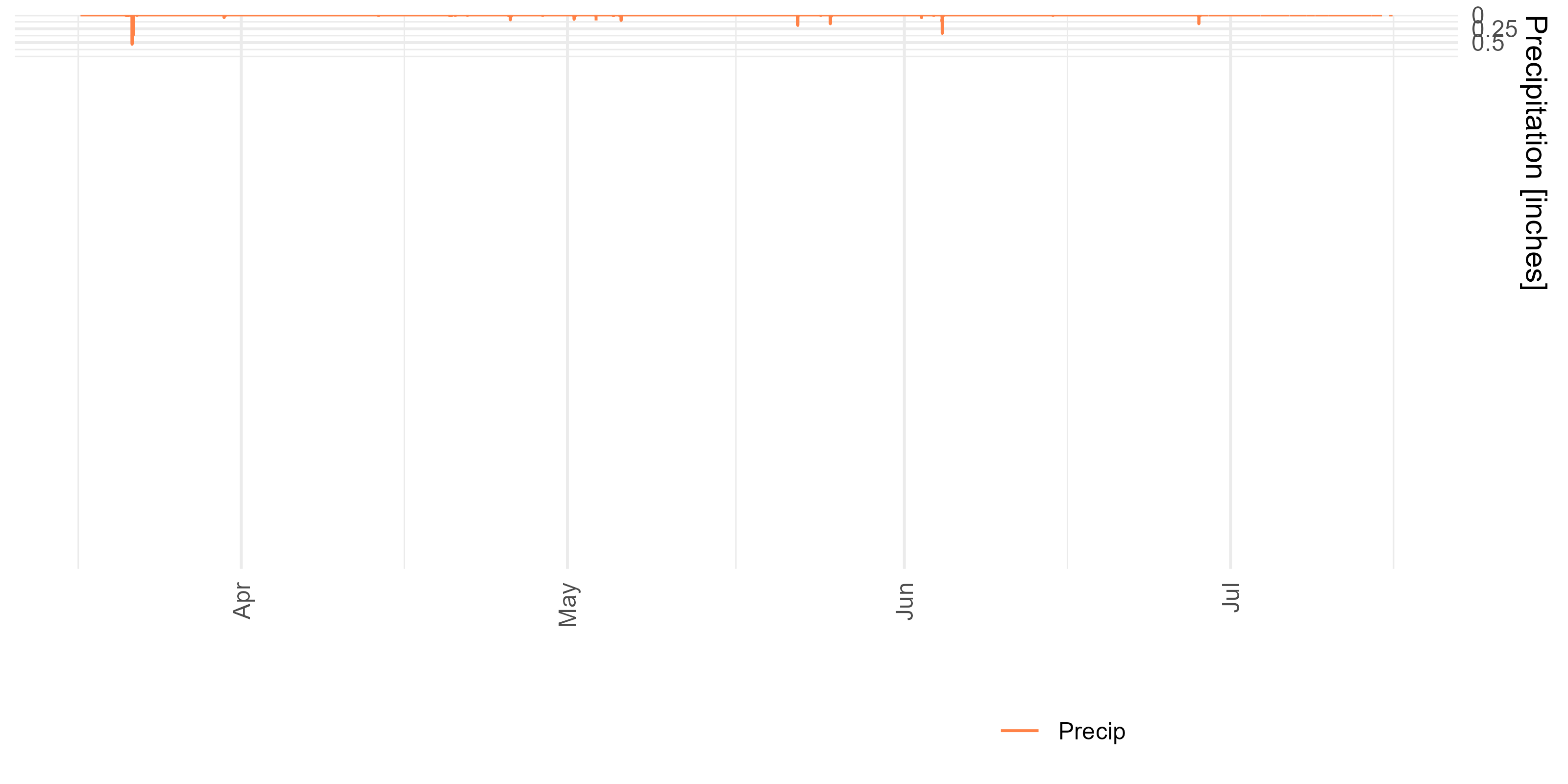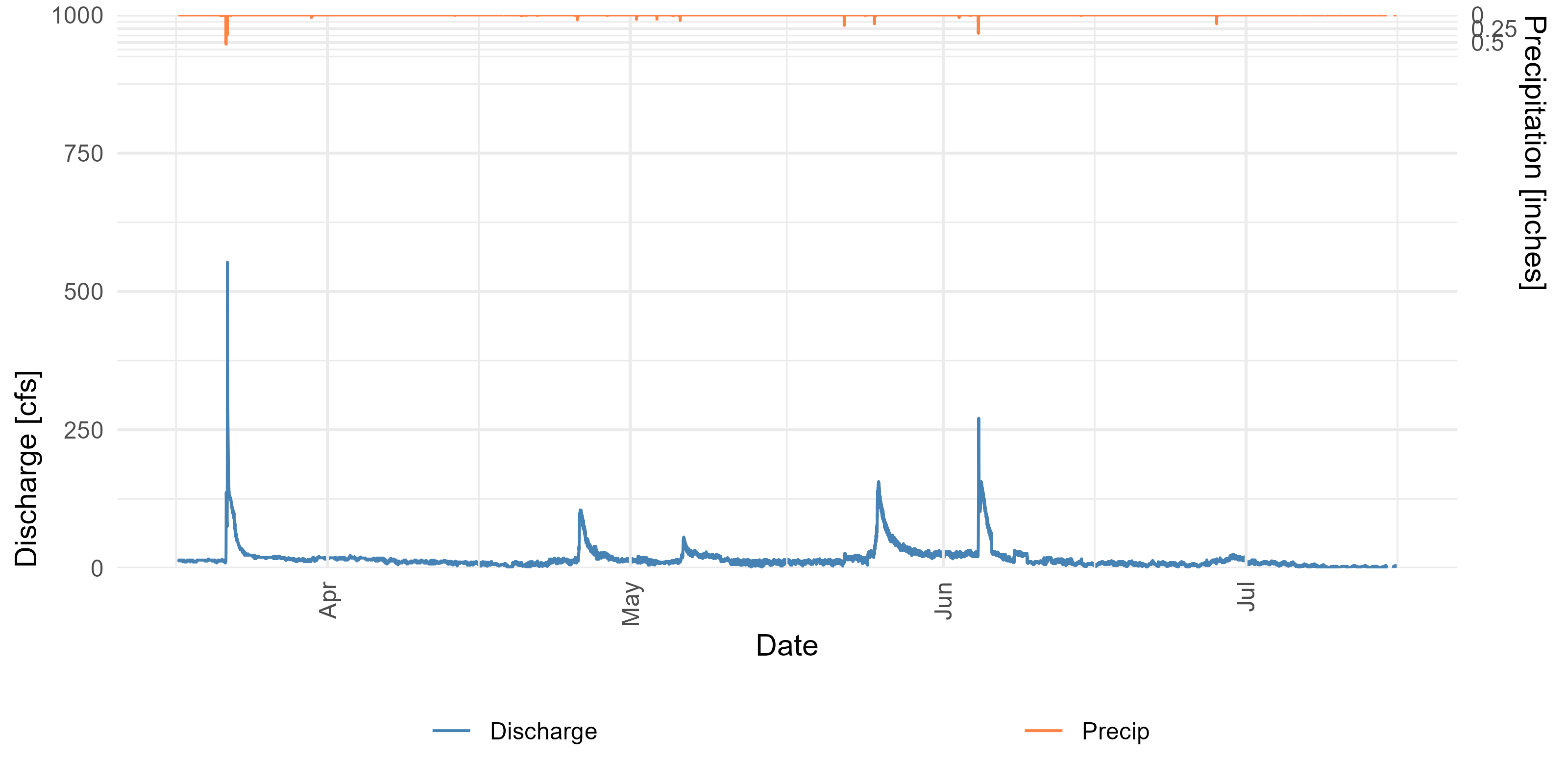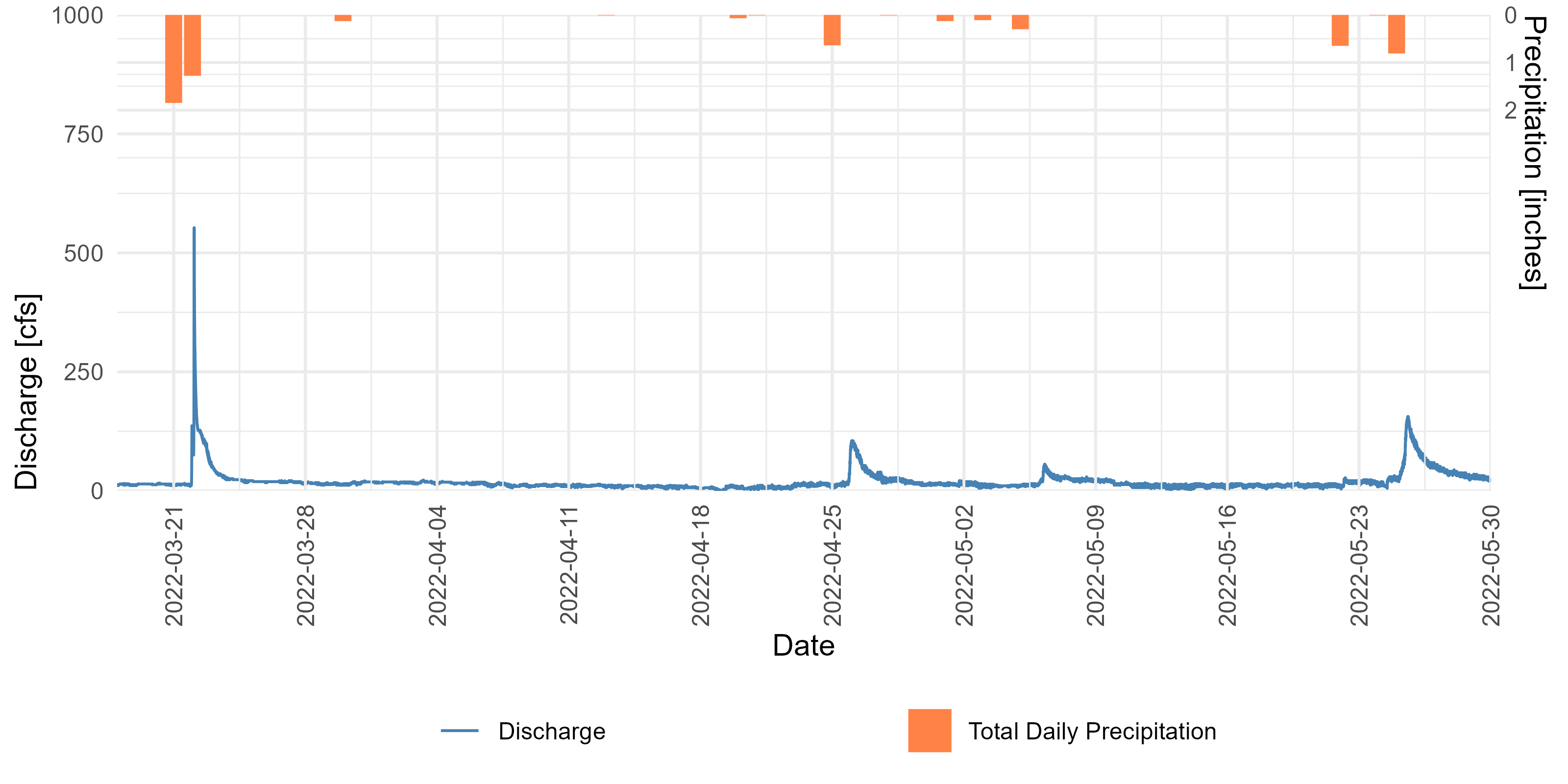# Combine a hydrograph and hyetograph in ggplot2

Streamflow hydrographs and precipitation hyetographs are commonly combined into one plot by hydrologists. Here is one way to make these plots in R.

Michael Schramm https://michaelpaulschramm.com (Texas Water Resources Institute)https://twri.tamu.edu
2022-07-15

It isn’t entirely uncommon to come across a streamflow hydrograph combined with a precipitation hyetograph. Although the general wisdom is to avoid combining data of different scales on one graph, this practice seems accepted in the hydrology commuinity, in particular with the precipitation represented along the top of the plot. I’m not one to judge.

Here is an approach to do it with ggplot and cowplot.

This example uses “instantaneous” discharge and precipitation data obtained from the USGS via the amazing dataRetrieval package.

``````library(dataRetrieval)
library(tidyverse)
library(lubridate)
library(cowplot)

site <- "08105872"

discharge <- readNWISuv(siteNumbers = site,
parameterCd = "00060",
startDate = "2022-03-17",
endDate = "2022-07-15")
discharge <- renameNWISColumns(discharge)

precip <- readNWISuv(siteNumbers = site,
parameterCd = "00045",
startDate = "2022-03-17",
endDate = "2022-07-15")
precip <-renameNWISColumns(precip)

## join the discharge and precip by date

joined_data <- discharge |>
left_join(precip |>  select(dateTime, Precip_Inst),
by = c("dateTime" = "dateTime"))``````

Streamflow is plotted normally using `ggplot()`. I’m am taking the time to move labels and legends where I want them in the final figure, so bear with me.

``````p1 <- ggplot(joined_data) +
geom_line(aes(dateTime, Flow_Inst, color = "Discharge")) +
scale_y_continuous(position = "left",
limits = c(0, 1000),
expand = c(0,0)) +
scale_color_manual(values = c("steelblue")) +
guides(x = guide_axis(angle = 90)) +
labs(y = "Discharge [cfs]",
x = "Date") +
theme_minimal() +
theme(axis.title.y.left = element_text(hjust = 0),
legend.position = "bottom",
legend.justification = c(0.25, 0.5),
legend.title = element_blank())
p1``````Precipitation will be plotted from the top down. Here, I’m using a line graph since it is the easiest way to start.

``````p2 <- ggplot(joined_data) +
geom_line(aes(dateTime, Precip_Inst, color = "Precip")) +
scale_y_reverse(position = "right",
limits = c(10,0),
breaks = c(0,0.25,0.5),
labels = c(0,0.25,0.5),
expand = c(0,0)) +
scale_color_manual(values = c("sienna1")) +
guides(x = guide_axis(angle = 90)) +
labs(y = "Precipitation [inches]", x = "") +
theme_minimal() +
theme(axis.title.y.right = element_text(hjust = 0),
legend.position = "bottom",
legend.justification = c(0.75, 0.5),
legend.title = element_blank())
p2``````Now we can use cowplot and the `align_plots()` function to combine things.

``````aligned_plots <- align_plots(p1, p2, align = "hv", axis = "tblr")
out <- ggdraw(aligned_plots[]) + draw_plot(aligned_plots[])
out``````This doesn’t technically include a hyetograph, which is a histogram of streamflows. It take a little more care to ensure the x-axis on both graphs line up if you want to aggregate precipitation. For simplicity, this example aggregates streamflow by day. Essentially, use the `scale_x_date()` and `scale_x_datetime()` functions to set common limits and breaks. This should adapt to most situations.

``````p1 <- p1 +
scale_x_datetime(date_breaks = "week",
limits = c(as.POSIXct("2022-03-18", tz="UT"),
as.POSIXct("2022-05-30", tz="UT")),
expand = c(0,0))

total_precip <- precip |>
mutate(date = as.Date(dateTime)) |>
group_by(date) |>
summarise(Precip = sum(Precip_Inst))

p3 <- ggplot(total_precip) +
geom_col(aes(date, Precip, fill = "Total Daily Precipitation")) +
scale_y_reverse(position = "right",
limits = c(10,0),
breaks = c(0,1,2),
labels = c(0,1,2),
expand = c(0,0)) +
scale_x_date(date_breaks = "week",
limits = c(as.Date("2022-03-18"),
as.Date("2022-05-30")),
expand = c(0,0)) +
scale_fill_manual(values = c("sienna1")) +
guides(x = guide_axis(angle = 90)) +
labs(y = "Precipitation [inches]", x = "") +
theme_minimal() +
theme(axis.title.y.right = element_text(hjust = 0),
legend.position = "bottom",
legend.justification = c(0.75, 0.5),
legend.title = element_blank())

aligned_plots <- align_plots(p1, p3, align = "hv", axis = "tblr")
ggdraw(aligned_plots[]) + draw_plot(aligned_plots[])``````### Corrections

If you see mistakes or want to suggest changes, please create an issue on the source repository.

### Reuse

Text and figures are licensed under Creative Commons Attribution CC BY 4.0. Source code is available at https://github.com/mps9506/mschramm, unless otherwise noted. The figures that have been reused from other sources don't fall under this license and can be recognized by a note in their caption: "Figure from ...".

### Citation

For attribution, please cite this work as

`Schramm (2022, July 15). @mpschramm: Combine a hydrograph and hyetograph in ggplot2. Retrieved from https://michaelpaulschramm.com/posts/2022-07-15-hydrograph/`

BibTeX citation

```@misc{schramm2022combine,
author = {Schramm, Michael},
title = {@mpschramm: Combine a hydrograph and hyetograph in ggplot2},
url = {https://michaelpaulschramm.com/posts/2022-07-15-hydrograph/},
year = {2022}
}```